# C program to find the length of a string

In this article, you will learn and get code about how to find and print the length of any given string given by the user (at run-time) using the following approaches:

## Print the length of a string using the strlen() function

To find the length of any given string in C programming, you have to ask the user to enter the string and then find its length using the strlen() function of the string.h library, as shown in the program given below:

```#include<stdio.h>
#include<conio.h>
#include<string.h>
int main()
{
char str;
int len;
printf("Enter the string: ");
gets(str);
len = strlen(str);
printf("\nLength of the string = %d", len);
getch();
return 0;
}```

As the above program was written in the Code::Blocks IDE, here is the first snapshot of the sample run: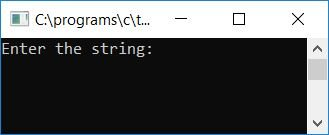Now supply any string, say "codescracker," and press the ENTER key to see the length of the given string as shown here in the second snapshot: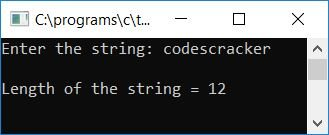The function named strlen() takes one argument as a string and returns the length. Therefore, the length of the string, say str, gets returned by the function strlen() and initialized to the variable len. Print the value of len as output.

## Find the length of a string without using the strlen() function

This program does not use the strlen() function to find the length; rather, the length gets calculated manually here:

```#include<stdio.h>
#include<conio.h>
int main()
{
char ch;
int count=0, i;
printf("Enter any string: ");
scanf("%s", ch);
for(i=0; ch[i]!='\0'; i++)
count++;
printf("\nLength of the String = %d", count);
getch();
return 0;
}```

Here is its sample run: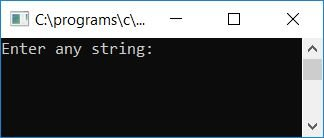Enter any string as input, for example, codescracker, and press the ENTER key to see its length as output: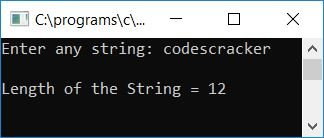As in the above program, the string was received from the user using the scanf() function. As a result, whenever the user enters a string that contains spaces, only the first word is received and the rest is skipped. Here is a sample run in which the user entered any string containing space, such as "codes cracker,"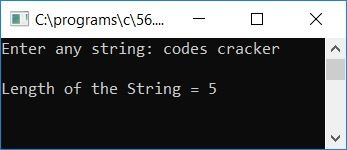As you can see, the word before the space is "codes," therefore the program will only calculate the length of "codes" that will be 5. To solve this problem, we have to use the gets() function in place of scanf() to receive the string (along with spaces) from the user, as shown in the program given below:

```#include<stdio.h>
#include<conio.h>
int main()
{
char str;
int count=0, i;
printf("Enter any string: ");
gets(str);
for(i=0; str[i]!='\0'; i++)
count++;
printf("\nLength of the String = %d", count);
getch();
return 0;
}```

Here is the final snapshot of the first sample run:Now let's take another sample run where the user enters a string that contains spaces, say "codes cracker." Here is the final snapshot of the second sample run: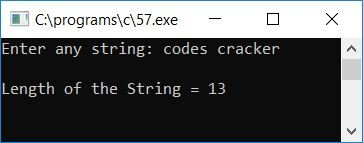#### Program Explained

• Receive any string as input using the gets() function.
• Use a for loop to start from the first character of the string to the last.
• If the null-terminated character ('\0') appears, it indicates that nothing is left in the string.
• Therefore, until the null-terminated character comes, increase the value of the count variable each and every time the program flow goes inside the for loop.
• The count variable is initialized to 0 at the beginning of the program.
• Therefore, from the first character to the last character of the string, the count variable will be incremented each and every time.
• It means that the count variable at last will hold the length of the string; that is, how many characters are present inside the string, say str.
• Finally, print the value of count that shows the length of the string.

## Find string length using a user-defined function

This program is made using user-defined functions to do the same job as the previous one:

```#include<stdio.h>
#include<conio.h>
int findStringLen(char str);
int main()
{
char str;
int len;
printf("Enter any string: ");
gets(str);
len = findStringLen(str);
printf("\nLength of the String = %d", len);
getch();
return 0;
}
int findStringLen(char s)
{
int i, count=0;
for(i=0; s[i]!='\0'; i++)
count++;
return count;
}```

Here is the final snapshot of the sample run: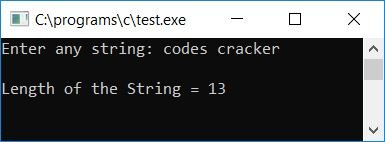#### The same program in different languages

C Quiz

« Previous Program Next Program »